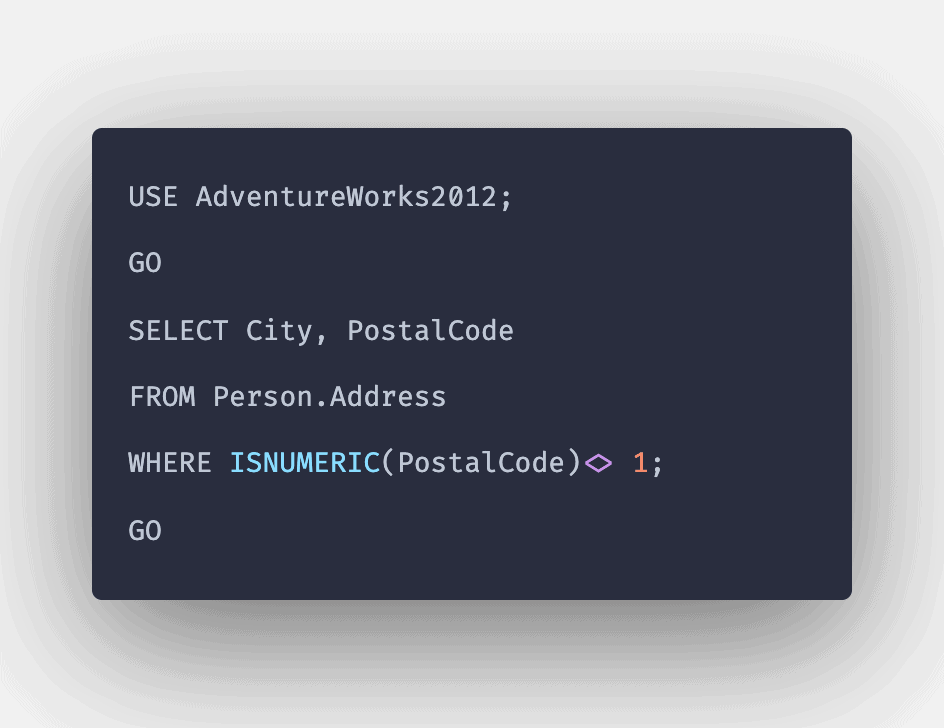# SQL ISNUMERIC() Function: Complete Guide

0
2SQL ISNUMERIC function tests whether the expression is numeric. The ISNUMERIC() function accepts an expression and returns 1 if it is a valid numeric type; otherwise, it returns 0.

## SQL ISNUMERIC()

SQL ISNUMERIC() is a built-in function that is used to check whether the expression passed as an input is the valid number or not. The ISNUMERIC() function returns 1 if the expression is a valid number. Otherwise, it returns 0.

### Syntax

```ISNUMERIC (Expression);
```

### Parameters

Expression: The expression must be checked whether it is a valid number.

The function checks whether the expression entered can be converted to a numeric datatype or not.

Note that a valid numeric type is one of the following:

Exact numbers: BIGINT, INT, SMALLINT, TINYINT, and BIT

Fixed precision: DECIMAL, NUMERIC

Approximate: FLOAT, REAL

Monetary values: MONEY, SMALLMONEY

### Query 1

```SELECT ISNUMERIC (9876);
```

```1
```

#### Explanation

The expression entered is already in the numeric form. So, the function returned 1 as an output.

### Query 2

```SELECT ISNUMERIC (‘9876’);
```

```1
```

#### Explanation

The expression was in string format, which returned a valid number when converted implicitly to numeric form. So, the function returned 1 as an output.

### Query 3

```SELECT ISNUMERIC (10*5);
```

`1`

#### Explanation

The arithmetic expression, when executed, returned a valid number. Thus, the function returned 1 as an output.

### Query 4

```SELECT ISNUMERIC (‘AppDividend.Com’);
```

`0`

#### Explanation

The expression was in string format, which, when tried to be converted implicitly to numeric form, didn’t return a valid number. So, the function returned 0 as an output.

### Query 5

```SELECT ISNUMERIC (‘2020-02-28’);
```

`0`

#### Explanation

The expression was in datetime format, which cannot be converted to numeric form. So, the function returned 0 as an output.

That’s it for this tutorial.